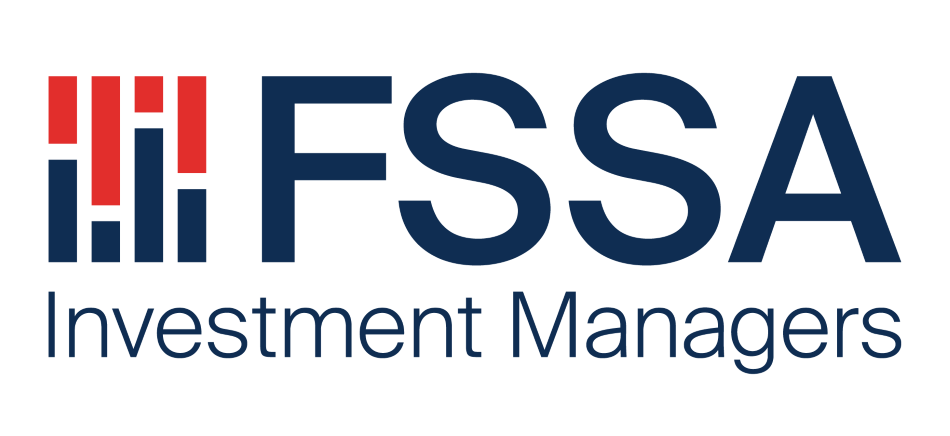Specialist in Asia Pacific, Japan, China, India and South East Asia and Global Emerging Market equities.

##### InsightsOur philosophy is very simple. We are constantly searching for high quality businesses and when we acquire them, we will work relentlessly with them to create long-term sustainable value through innovation, ESG-led and proactive asset management.

Investment strategies

InsightsLeader in systematic equities across market cap weighted indices, smart beta and active quantitative strategies

##### InsightsAt Stewart Investors, we believe in putting people first. Our investment world-view is of a series of partnerships – with each other, with our clients, with the companies we invest in, the people who buy their goods and services, and with the wider society in which we all live and work.

# YFYS Simulations

## Assumptions

We impose some high-level assumptions on the asset allocation bands of a balanced fund (default MySuper option). These assumptions are largely consistent with the assumptions provided by the modeling conducted by the Conexus working group. We have used 7 asset classes, and for simplicity without a loss of generality, we have ignored sector tilts such as broad caps vs small caps or developed vs. emerging markets. This is also consistent with APRA’s benchmarking, which does not distinguish for small caps or emerging markets.

Table 1 shows our mean SAA assumptions for the 7 asset classes and our asset allocation band deviation assumptions. Asset allocation deviations can be due to changes to a fund’s SAA or shorter term TAA / DAA positioning. In these simulations below, we don’t make further assumptions for distinguishing between the two with AA deviations. That is, we assume fund risk comes from three sources: i) SAA, ii) AA deviations and iii) manager selection. However, we note that AA deviations due to changes in long term SAA will be incorporated in the APRA benchmarks, and thus not assessed for under-performance, but shorter term TAA will be assessed.

Table 1. Generic Asset Allocation Bands for MySuper default option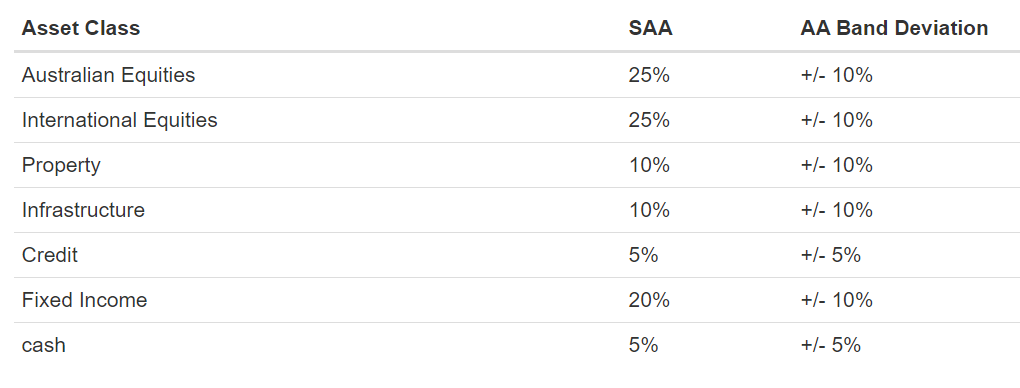We make some long-term mean variance assumptions. These assumptions are based on long term views of growth assets, mid-risk assets and defensive assets.

Table 2. Asset class mean variance assumptions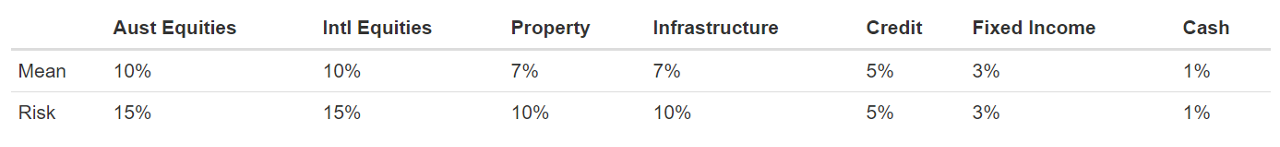We have simplified asset class correlations into the following five categories.

• Growth assets: Equities
• Mid assets: Property and Infrastructure
• Credit
• Govt Bonds (FI)
• Cash

We assume asset classes with categories, such as Australian vs International Equities, have a correlation of 0.8.

Table 3. Asset class correlation matrix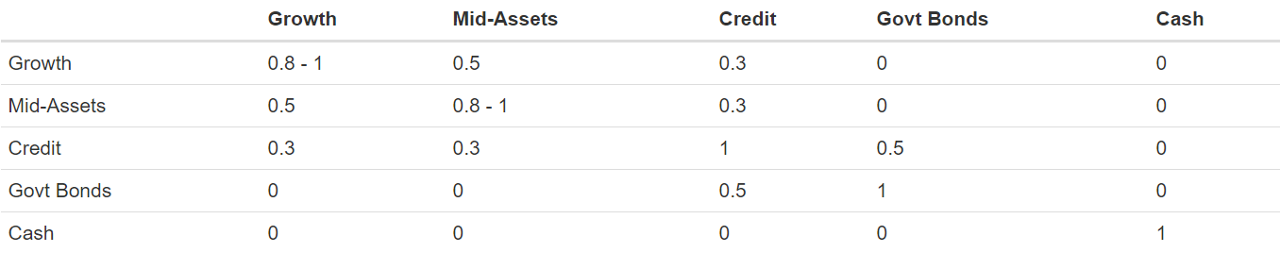Furthermore, we have made some assumptions on manager selection tracking error. We have assumed no correlation between manager alpha and asset class beta, i.e. alpha is orthogonal to beta. We have assumed a small level of correlation between cross-asset manager alpha, between say Australian Equities and International Equities.

Table 4. Generic Manager Tracking Error Assumptions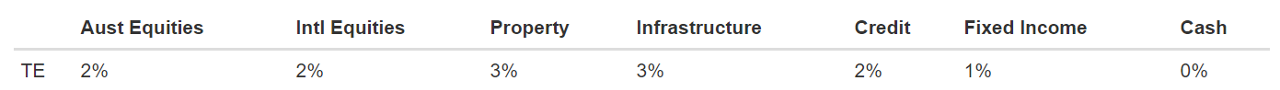YFYS Simulations
###########################
# Assumptions: Fund Weights and Capital Markets
###########################

# Generic MySuper fund assumptions
#                           weights, weight deviations, mgr tracking error
bfAssumptions <- as.data.frame(rbind(c(0.25, 0.10, 0.02),        # AusEq
c(0.25, 0.10, 0.02),        # IntEq
c(0.10, 0.10, 0.03),        # Prop
c(0.10, 0.10, 0.03),        # Infra
c(0.05, 0.05, 0.02),        # Credit
c(0.20, 0.10, 0.01),        # Fixed Income
c(0.05, 0.05, 0.00)))       # Cash
rownames(bfAssumptions) <- c("Aust-Equities", "Intl-Equities", "Property", "Infrastructure", "Credit", "Fixed-Income", "cash")
colnames(bfAssumptions) <- c("meanWts", "devWts", "mgrTE")
# generate upper and lower asset class bounds
bfAssumptions$upper <- bfAssumptions[,'meanWts'] + bfAssumptions[,'devWts'] bfAssumptions$lower <- bfAssumptions[,'meanWts'] - bfAssumptions[,'devWts']
# number of assets in our model
numAssets <- nrow(bfAssumptions)

# General asset class assumptions
#                  AusEq, IntEq, Prop, Infra, Credit, FI and Cash
# mean
meanAsset      <- c(0.10, 0.10,  0.07, 0.07,  0.05,  0.03,  0.01)
# standard deviation
volAsset       <- c(0.15, 0.15,  0.10, 0.10,  0.05,  0.03,  0.01)
# correlation
corAsset <- rbind(c( 1.0,  0.8,   0.5,  0.5,   0.3,   0.0,  0.0),
c( 0.8,  1.0,   0.5,  0.5,   0.3,   0.0,  0.0),
c( 0.5,  0.5,   1.0,  0.8,   0.3,   0.0,  0.0),
c( 0.5,  0.5,   0.8,  1.0,   0.3,   0.0,  0.0),
c( 0.3,  0.3,   0.3,  0.3,   1.0,   0.5,  0.0),
c( 0.0,  0.0,   0.0,  0.0,   0.5,   1.0,  0.0),
c( 0.0,  0.0,   0.0,  0.0,   0.0,   0.0,  1.0))

# Manager selection assumptions
# assume zero correlation between mgr alpha and asset class beta
# assume some small levels of correlation between cross-asset mgr alpha
corMgr <-   rbind(c( 1.0,  0.5,   0.0,  0.0,   0.0,   0.0,  0.0),
c( 0.5,  1.0,   0.0,  0.0,   0.0,   0.0,  0.0),
c( 0.0,  0.0,   1.0,  0.3,   0.0,   0.0,  0.0),
c( 0.0,  0.0,   0.3,  1.0,   0.0,   0.0,  0.0),
c( 0.0,  0.0,   0.0,  0.0,   1.0,   0.3,  0.0),
c( 0.0,  0.0,   0.0,  0.0,   0.3,   1.0,  0.0),
c( 0.0,  0.0,   0.0,  0.0,   0.0,   0.0,  1.0))

# Build model covariance matrix
# covariance matrix has three components: SAA, AA deviations, and Manager Selection
covAsset <- volAsset %*% t(volAsset) * corAsset
covMgr <- bfAssumptions[,"mgrTE"] %*% t(bfAssumptions[,"mgrTE"]) * corMgr
covAssetMgr <- matrix(0, numAssets, numAssets)

covAssetMgr <- rbind(cbind(covAsset, covAsset, covAssetMgr),
cbind(covAsset, covAsset, covAssetMgr),
cbind(covAssetMgr, covAssetMgr,covMgr))

colnames(covAssetMgr) <- c(paste0(rownames(bfAssumptions), " SAA"), paste0(rownames(bfAssumptions), " AA Deviations"), paste0(rownames(bfAssumptions)," Mgr Selection"))
rownames(covAssetMgr) <- colnames(covAssetMgr)

## Internal Functions

Here lies some internal functions we will use for our analysis.

###########################
# Functions
###########################

# Generate a random MySuper balanced fund
# check weights add to 1 and satisfies constraints
.genBalancedFund <- function(bfAssumptions) {
wts <- apply(bfAssumptions, 1, function(x) { runif(1, min = x, max = x) })
wts <- wts / sum(wts)
# check to see if weights satisfy lower-upper bounds
if(sum(bfAssumptions$lower < wts) == nrow(bfAssumptions) && sum(bfAssumptions$upper > wts) == nrow(bfAssumptions))
return(wts)
else
.genBalancedFund(bfAssumptions)
}

# Calculate Marginal Contribution to Total Risk and Tracking Error
# notes: http://webuser.bus.umich.edu/ppasquar/shortpaper6.pdf
calcMarginalRisk <- function() {
fundWgts <- .genBalancedFund(bfAssumptions)
TE <- sapply(sims, function(x) x$mcTE) rownames(TR) <- colnames(covAssetMgr) rownames(TE) <- colnames(covAssetMgr) # average marginal contribution to risk mcTable <- data.frame(Marginal Contribution to Total Risk (%) = round(apply(TR, 1, mean)*100, 2), Marginal Contribution to Tracking Error (bps) = round(apply(TE, 1, mean)*10000,2)) kable(mcTable, caption = "Marginal Contributions to Risk")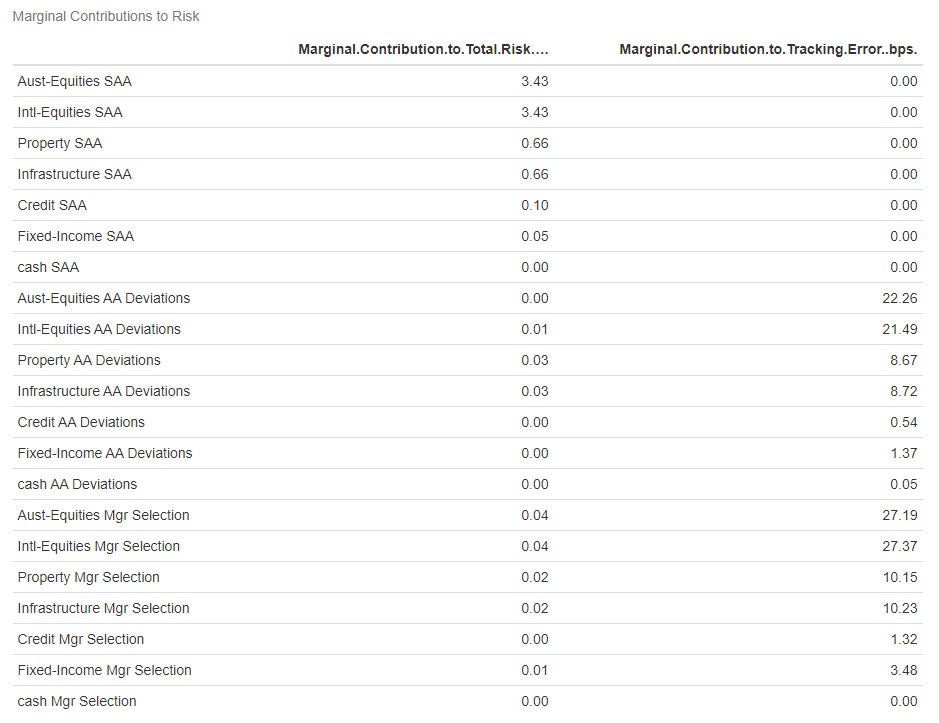# % of the marginal contribution from SAA, AA deviations and Manager Selection row.idx <- lapply(c("SAA", "AA Deviations", "Mgr Selection"), function(x) grep(x, rownames(TE))) pctTR <- apply(TR, 2, function(x) sapply(row.idx, function(y) sum(x[y]) / sum(x))) pctTE <- apply(TE, 2, function(x) sapply(row.idx[-1], function(y) sum(x[y]) / sum(x))) rownames(pctTR) <- c("SAA", "AA Deviations", "Mgr Selection") rownames(pctTE) <- c("AA Deviations", "Mgr Selection") # plot the distributions # marginal contributions of total risk pctTR_l <- melt(t(pctTR)) colnames(pctTR_l) <- c("sims", "source", "contribution") ggplot(pctTR_l, aes(x = contribution *100, fill = source)) + geom_histogram(aes(y=..density..), bins = 100) + geom_density(alpha=.2) + facet_free(source~. , scales = "free") + xlab("Percentage contribution to Total Risk (%)") + theme_classic() + ggtitle("The Percentage Marginal Contribution of Total Risk")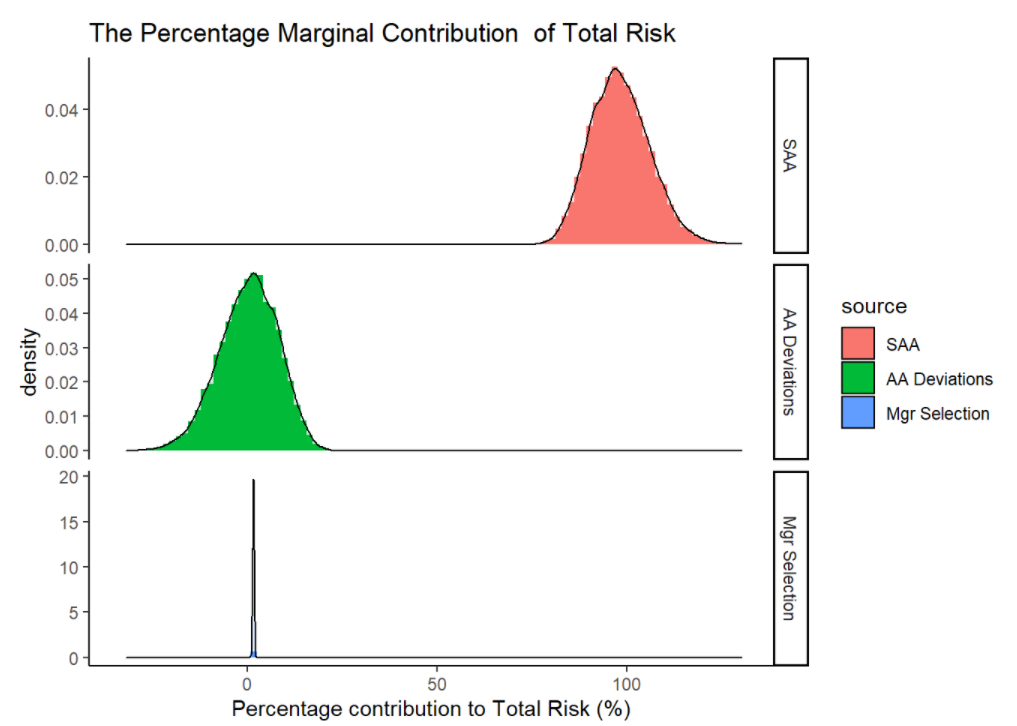# marginal contributions of tracking error pctTE_l <- melt(t(pctTE)) colnames(pctTE_l) <- c("sims", "source", "contribution") ggplot(pctTE_l, aes(x = contribution *100, fill = source)) + geom_histogram(aes(y=..density..), bins = 100) + geom_density(alpha=.2) + facet_free(source~. , scales = "free") + xlab("Percentage contribution to Tracking Error (%)") + theme_classic() + ggtitle("The Percentage Marginal Contribution of Tracking Error (Peer Risk)")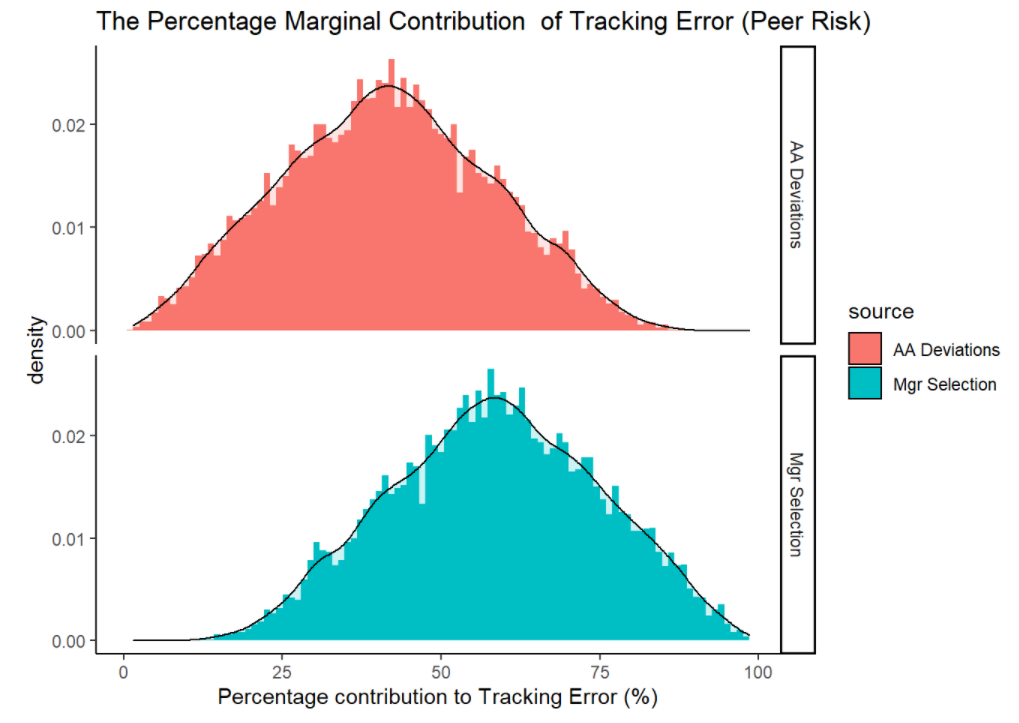## Modeling 2. On the probabilities of meeting member retirement outcomes Here, we examine the impact of asset allocation and manager selection in meeting member retirement outcomes. We make a simplistic assumption that a member meets their retirement outcomes if they are able to accumulate their contributions under an annual return of CPI + 4% consistently. For instance, a women earning$100,000 pa with 2% wage growth and 9.5% super contributions over a period of 20 years should accumulate up to $441,975 for her super. Let’s assume this to be the retirement goal. We look at the probability our ‘generic balanced plans’ misses these objectives. • Manager selection is not a key driver of member outcomes. • Asset allocation drives whether a member meets its retirement outcomes. # sample member information salary <- 100000L annualContributions <- salary * 0.095 wageGrowth <- 0.02 yearsToRetirement <- 20 contributions <- annualContributions * cumprod(rep(1+wageGrowth, yearsToRetirement)) # CPI + 4% benchmark # where CPI assumed to be circa 2% pa bench <- rep(0.06, length(contributions)) cumbench <- cumprod(1 + bench) accumbench <- sum(contributions * rev(cumbench)) print(paste0("For an annual salary of ", salary, " with 9.5% super contribution and wage growth of ", wageGrowth, " over ", yearsToRetirement, " years, the benchmark accumulation is: ", accumbench)) ##  "For an annual salary of 100000 with 9.5% super contribution and wage growth of 0.02 over 20 years, the benchmark accumulation is: 441975.280155874" # simulate retirement outcomes under 3 manager selection scenarios nsims <- 10000 outcomes <- list() outcomes[["none"]] <- sapply(1:nsims, function(x) calcRetirement(contributions)) outcomes[["negative"]] <- sapply(1:nsims, function(x) calcRetirement(contributions, rep(-0.005, numAssets))) outcomes[["positive"]] <- sapply(1:nsims, function(x) calcRetirement(contributions, rep(+0.005, numAssets))) outcomes <- as.data.frame(outcomes) pctOutcomes <- outcomes / accumbench - 1 # plot simulations pctOutcomes_long <- gather(pctOutcomes, alpha, outcome, 1:3) ggplot(pctOutcomes_long, aes(x = outcome * 100, fill = alpha)) + geom_histogram(aes(y=..density..), bins=50) + geom_density(alpha=.2) + facet_free(alpha~., scales = "free_y") + geom_vline(aes(xintercept=mean(pctOutcomes$positive)*100, col="red"), show.legend = F) +
geom_vline(aes(xintercept=mean(pctOutcomes$none)*100, col="green"), show.legend = F) + geom_vline(aes(xintercept=mean(pctOutcomes$negative)*100, col="blue"), show.legend = F) +
xlab("Percentage over the CPI + 4 benchmark at end of accumulation") + theme_classic() +
ggtitle("The Distribution of Member Outcomes")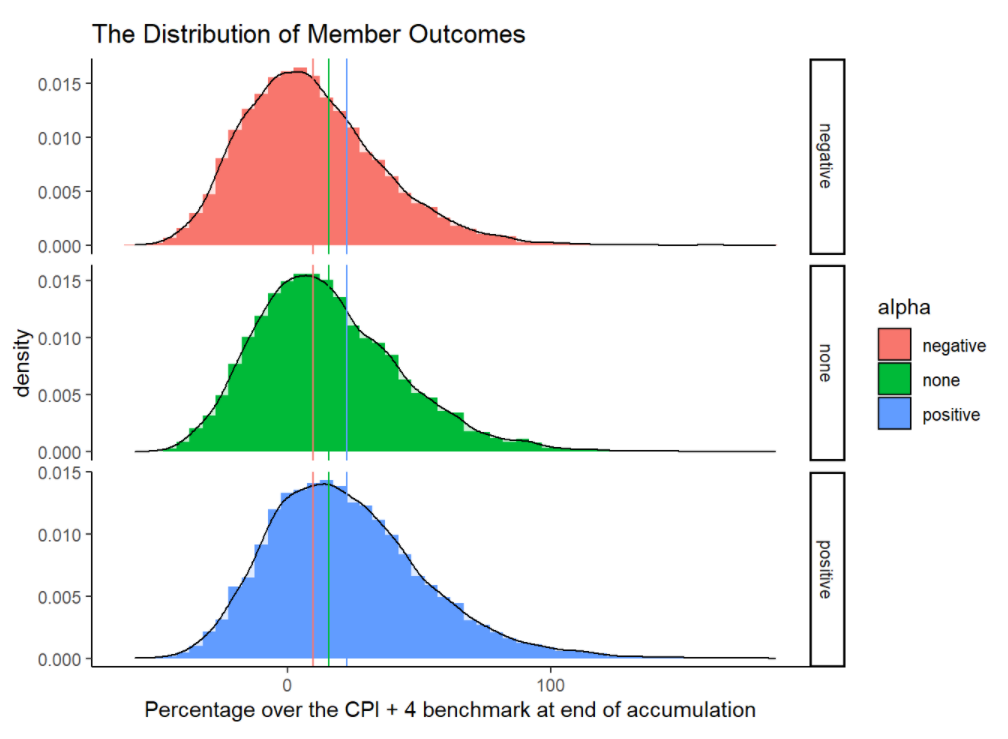# percentage of outcomes that underperformed the CPI+4% benchmark
apply(pctOutcomes, 2, function(x) sum(x< 0) / length(x) )
##     none negative positive
##   0.3107   0.3952   0.2347

## Modeling 3. The Probability of Failure increases with Tracking Error

In this section, we examine how the annual performance test may incentivize index herding. We find that the probability of failure is reduced if funds choose to take less tracking error to the APRA benchmark returns. We show that at lower levels of tracking error, poor performance cannot be detected.

trackingErrors <-seq(0, 0.10, 0.001)
informationRatios <- seq(-0.5, +0.5, 0.01)

results <- matrix(0, length(trackingErrors), length(informationRatios))
rownames(results) <- trackingErrors * 100
colnames(results) <- informationRatios
for (i in 1:length(trackingErrors)) {
results[i, ] <- sapply(informationRatios, function(x) .pFailHurdle(x, TE = trackingErrors[i], years = 8))
}

levelplot(results,ylab = "Information Ratio", xlab = "Tracking Error (%)",
main = "Probability of Failure",
scales=list(x = list(at=seq(1, nrow(results),10)), y = list(at=seq(1, ncol(results),10))),
col.regions = colorRampPalette(rev(brewer.pal(4, "RdBu"))))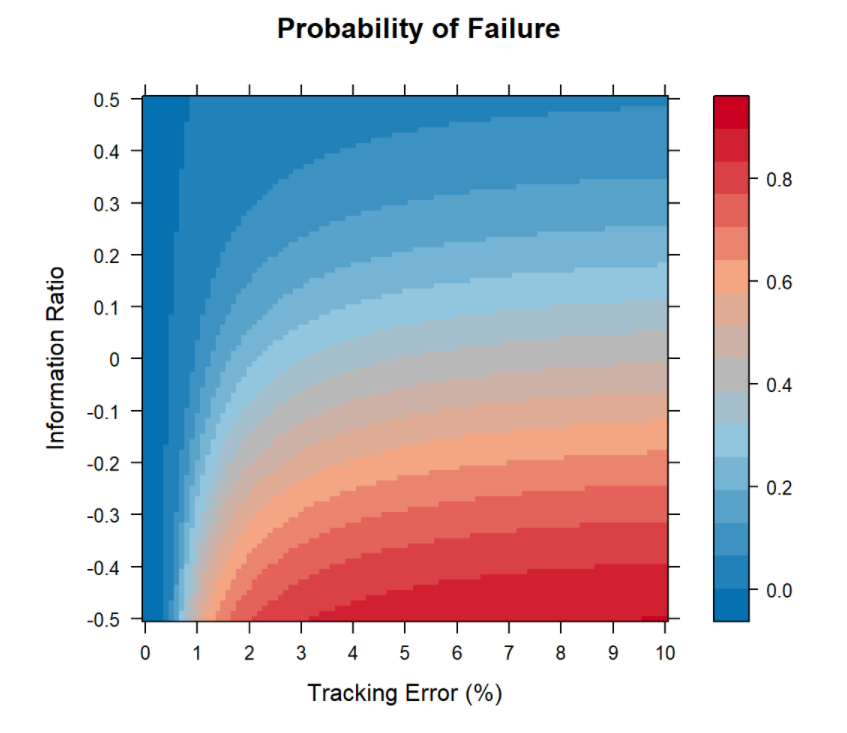## Modeling 4. Impact of Time Frame (Assessment Length) on False Positives

We test to examine if 8 years enough to assess a good fund from a bad fund. We find that at 8 years, most super funds would need to run at tracking errors of circa 1% or lower to be reasonably confident in not being accidently tested as an underperforming fund.

years <- seq(1,20, 1)
trackingErrors <- seq(0, 0.10, 0.005 )

results <- matrix(0, length(trackingErrors), length(years))
rownames(results) <- trackingErrors * 100
colnames(results) <- years
for (i in 1:length(trackingErrors)) {
results[i, ] <- sapply(years, function(x) .pFailHurdle(IR = 0, TE = trackingErrors[i], years = x))
}

levelplot(results,ylab = "Years", xlab = "Tracking Error (%)",
main = "Probability of Failure",
col.regions = colorRampPalette(rev(brewer.pal(4, "RdBu"))))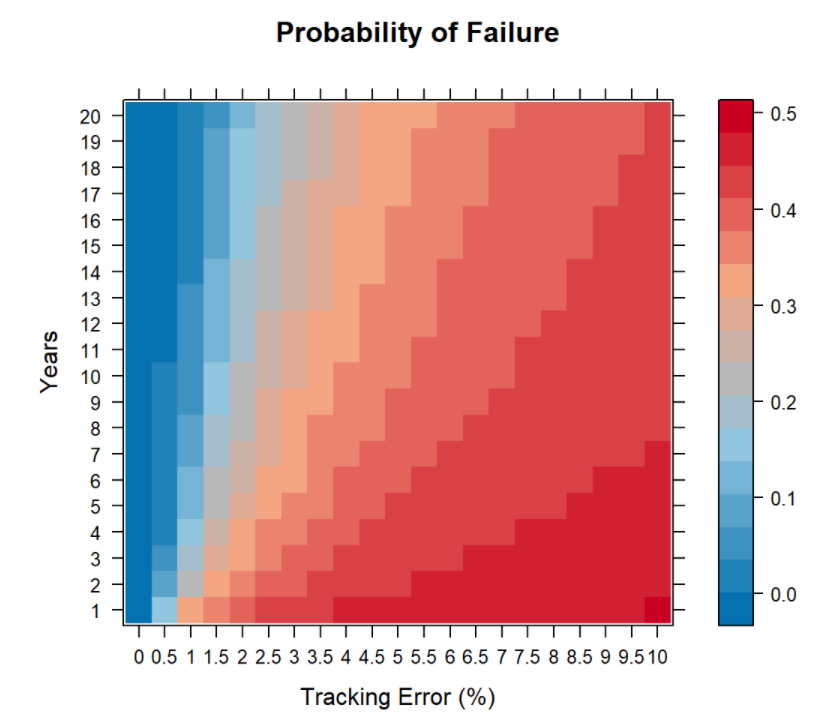## Disclaimer

This Workbook has been prepared by First Sentier Investors Realindex Pty Limited (ABN 24 133 312 017, AFSL 335381) (FSI RP) as a supplement to the publication titled “Addressing the Underperformance in Superannuation: Concerns around the Your Future, Your Super proposal” (Publication) and should only be read together with the Publication. The purpose of this Workbook is to illustrate the assumptions and the basis for the calculations that have been summarised in the Publication. It is not intended to provide financial product advice. The information, assumptions and the basis for the calculations herein are in the opinion of FSI RP accurate, reliable and reasonable, however neither FSI RP, Mitsubishi UFJ Financial Group, Inc (MUFG) nor their respective affiliates offer any warranty that such assumptions will eventuate or that the information or calculations contain no factual errors. To the extent permitted by law, no liability is accepted by MUFG, FSI RP nor their respective affiliates for any loss or damage as a result of any reliance on the information, assumptions or calculations in this Workbook.